# Samacheer Kalvi Books: Tamilnadu State Board Text Books Solutions

## Samacheer Kalvi 10th Science Chapter 6 Nuclear Physics Notes PDF Download: Tamil Nadu STD 10th Science Chapter 6 Nuclear Physics NotesSamacheer Kalvi 10th Science Chapter 6 Nuclear Physics Notes PDF Download: Tamil Nadu STD 10th Science Chapter 6 Nuclear Physics Notes

Samacheer Kalvi 10th Science Chapter 6 Nuclear Physics Notes PDF Download: Students of class can download the Samacheer Kalvi 10th Science Chapter 6 Nuclear Physics Notes PDF Download from our website. We have uploaded the Samacheer Kalvi 10th Science Chapter 6 Nuclear Physics notes according to the latest chapters present in the syllabus. Download Samacheer Kalvi 10th Science Chapter 6 Nuclear Physics Chapter Wise Notes PDF from the links provided in this article.

## Samacheer Kalvi 10th Science Chapter 6 Nuclear Physics Notes PDF Download

We bring to you specially curated Samacheer Kalvi 10th Science Chapter 6 Nuclear Physics Notes PDF which have been prepared by our subject experts after carefully following the trend of the exam in the last few years. The notes will not only serve for revision purposes, but also will have several cuts and easy methods to go about a difficult problem.

 Board Tamilnadu Board Study Material Notes Class Samacheer Kalvi 10th Science Subject 10th Science Chapter Chapter 6 Nuclear Physics Format PDF Provider Samacheer Kalvi Books

## How to Download Samacheer Kalvi 10th Science Chapter 6 Nuclear Physics Notes PDFs?

2. Click on the Samacheer Kalvi 10th Science Notes PDF.
3. Look for your preferred subject.
4. Now download the Samacheer Kalvi 10th Science Chapter 6 Nuclear Physics notes PDF.

## Download Samacheer Kalvi 10th Science Chapter 6 Nuclear Physics Chapterwise Notes PDF

Students can download the Samacheer Kalvi 10th Science Chapter 6 Nuclear Physics Notes PDF from the links provided in this article.

### Samacheer Kalvi 10th Science Nuclear Physics Textual Solved Problems

10th Science Nuclear Physics Question 1.
Identify A, B, C, and D from the following nuclear reactions.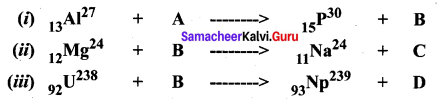Solution: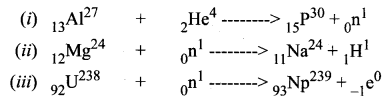A is alpha particle, B is neutron, C is proton and D is electron.

Nuclear Physics 10th Class Question 2.
A radon specimen emits radiation of 3.7 × 103 GBq per second. Convert this disintegration in terms of a curie, (one curie = 3.7 × 1010 disintegration per second)
Solution:
1 Bq = one disintegration per second
one curie = 3.7 × 1010 Bq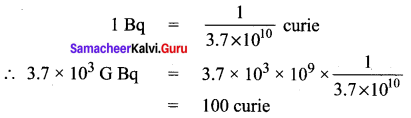Nuclear Physics Class 10 Question 3.
92U235 experiences one α – decay and one β – decay. Find the number of neutrons in the final daughter nucleus that is formed.
Solution:
Let X and Y be the resulting nucleus after the emission of the alpha and beta particles respectively.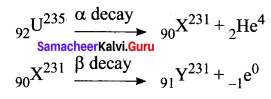Number of neutrons = Mass number – Atomic number = 231 – 91 = 140.

10th Nuclear Physics Question 4.
Calculate, the amount of energy released when a radioactive substance undergoes fusion and results in a mass defect of 2 kg.
Solution:
Mass defect in the reaction (m) = 2 kg
Velocity of light (c) = 3 × 108 ms-1
By Einstein’s equation,
Energy released E = mc2
= 2 × (3 × 108)2
= 1.8 × 1017 J.

### Samacheer Kalvi 10th Science Nuclear Physics Textual Evaluation

I. Choose the correct answer

Activity 6.1 Class 10 Science Question 1.
Man – made radioactivity is also known as _____.
(d) (a) & (c).
(d) (a) & (c).

Class 10 Science Chapter 6 Solutions Question 2.
Unit of radioactivity is:
(a) roentgen
(b) curie
(c) becquerel
(d) all the above
(d) all the above

Nuclear Physics Question 3.
Artificial radioactivity was discovered by _____.
(a) Becquerel
(b) Irene Curie
(c) Roentgen
(d) Neils Bohr.
(b) Irene Curie

Physics 10th Question 4.
In which of the following, no change in mass number of the daughter nuclei takes place:
(i) a decay;
(ii) P decay
(iii) y decay
(iv) neutron decay
(a) (i) is correct
(b) (ii) and (iii) are correct
(c) (i) and (iv) are correct
(d) (ii) and (iv) are correct
(b) (ii) and (iii) are correct

Question 5.
_____ isotope is used for the treatment of cancer.

Question 6.
Gamma radiations are dangerous because:
(a) it affects eyes and bones
(b) it affects tissues
(c) it produces genetic disorder
(d) it produces an enormous amount of heat
(c) it produces genetic disorder

Question 7.
_____ aprons are used to protect us from gamma radiations.
(b) Iron
(d) Aluminium.

Question 8.
Which of the following statements is / are correct?
(i) α particles are photons
(ii) Penetrating power of γ radiation is very low
(iii) Ionization power is maximum for α rays
(iv) Penetrating power of γ radiation is very high
(a) (i) & (ii) are correct
(b) (ii) & (iii) are correct
(c) (iv) only correct
(d) (iii) & (iv) are correct.
(d) (iii) & (iv) are correct.

Question 9.
Proton-Proton chain reaction is an example of:
(a) Nuclear fission
(b) α – decay
(c) Nuclear fusion
(d) β – decay
(c) Nuclear fusion

Question 10.
In the nuclear reaction X126⟶α decay zYA, the value of A & Z.
(a) 8, 6
(b) 8, 4
(c) 4, 8
(d) cannot be determined with the given data.
(c) 4, 8

Question 11.
Kamini reactor is located at _____.
(a) Kalpakkam
(b) Koodankulam
(c) Mumbai
(d) Rajasthan.
(a) Kalpakkam

Question 12.
Which of the following is/are correct?
(i) Chain reaction takes place in a nuclear reactor and an atomic bomb.
(ii) The chain reaction in a nuclear reactor is controlled.
(iii) The chain reaction in a nuclear reactor is not controlled.
(iv) No chain reaction takes place in an atom bomb.
(a) (i) only correct
(b) (i) & (ii) are correct
(c) (iv) only correct
(d) (iii) & (iv) are correct
(b) (i) & (ii) are correct

II. Fill in the blanks

Question 1.
One roentgen is equal to ______ disintegrations per second?
3.7 × 1010.

Question 2.
Positron is an _____.
antiparticle of electron.

Question 3.
Anaemia can be cured by _____ isotope.

Question 4.
Abbreviation of ICRP _____.
International Commission on Radiological Protection.

Question 5.
_____ is used to measure the exposure rate of radiation in humans.
Roentgen.

Question 6.
_____ has the greatest penetration power.
Gamma ray.

Question 7.
zYA→Z+1YA+X; Then X is _____.
−1e0 (β decay).

Question 8.
zXA→YAZ This reaction is possible in _____ decay.
Gamma (γ).

Question 9.
The average energy released in each fusion reaction is about _____ J.
3.84 × 10-12.

Question 10.
Nuclear fusion is possible only at an extremely high temperature of the order of _____ K.
107 to 109.

Question 11.
The radioisotope of _____ helps to increase the productivity of crops.
phosphorous (P – 32).

Question 12.
If radiation exposure is 100 R, it may cause _____.
fatal disease.

III. State whether the following statements are true or false: If false, correct the statement

Question 1.
Plutonium -239 is a fissionable material.
True.

Question 2.
Elements having an atomic number greater than 83 can undergo nuclear fusion.
False.
Correct Statement: Elements having an atomic number greater than 83 can undergo nuclear fusion.

Question 3.
Nuclear fusion is more dangerous than nuclear fission.
False.
Correct Statement: Nuclear fission is more dangerous than nuclear fusion. Because the average energy released in fission (3.2 × 10-11 J) process is more than the average energy released in fusion (3.84 × 10-12 J).

Question 4.
Natural uranium U-238 is the core fuel used in a nuclear reactor.
False.
Correct Statement: U-238 is not a fissile material but are abundant in nature. But in a reactor, this can be converted into a fissile material Pu239 and U233. Only fissile materials are used in the fuel of a nuclear reactor.

Question 5.
If a moderator is not present, then a nuclear reactor will behave like an atom bomb.
True.

Question 6.
During one nuclear fission on an average, 2 to 3 neutrons are produced.
True.

Question 7.
Einstein’s theory of mass-energy equivalence is used in nuclear fission and fusion.
True.

IV. Match the following

Question 1.

 1. BARC (a) Kalpakkam 2. India’s first atomic power station (b) Apsara 3. IGCAR (c) Mumbai 4. The first nuclear reactor in India (d) Tarapur

1. (c) Mumbai
2. (d) Tarapur
3. (a) Kalpakkam
4. (b) Apsara

Question 2.

 1. Fuel (a) lead 2. Moderator (b) heavy water 3. Coolant (c) Graphite 4. Shield (d) Uranium

1. (d) uranium
2. (c) Graphite
3. (b) heavy water

Question 3.

 1. Soddy Fagan (a) Natural radioactivity 2. Irene Curie (b) Displacement law 3. Henry Becquerel (c) Mass energy equivalence 4. Albert Einstein (d) Artificial Radioactivity

1. (b) Displacement law
2. (d) Artificial Radioactivity
3. (a) Natural radioactivity
4. (c) Mass energy equivalence

Question 4.

 1. Uncontrolled fission Reaction (a) Hydrogen Bomb 2. Fertile material (b) Nuclear Reactor 3. Controlled fission Reaction (c) Breeder reactor 4. Fusion reaction (d) Atom bomb

1. (d) Atom bomb
2. (c) Breeder reactor
3. (b) Nuclear Reactor
4. (a) Hydrogen Bomb

Question 5.

 1. Co – 60 (a) Age of fossil 2. I – 13 (b) Function of Heart 3. Na – 24 (c) Leukaemia 4. C – 14 (d) Thyroid disease

1. (c) Leukemia
2. (d) Thyroid disease
3. (b) Function of Heart
4. (a) Age of fossil

V. Arrange the following in the correct sequence

Question 1.
Arrange in descending order, on the basis of their penetration power.

1. Alpha rays
2. Beta rays
3. Gamma rays
4. Cosmic rays.

1. Gamma rays
2. Beta rays
3. Alpha rays
4. Cosmic rays.

Question 2.
Arrange the following in the chronological order of discovery.

1. A nuclear reactor
4. Discovery of radium.

2. Discovery of radium (1898)
3. Artificial radioactivity (1934)
4. Nuclear reactor (1942).

VI. Use the analogy to fill in the blank

Question 1.
Spontaneous process : Natural Radioactivity, Induced process: _____.
(or)
Man – made activity.

Question 2.
Nuclear Fusion : Extreme temperature, Nuclear Fission: _____.
Room temperature.

Question 3.
Increasing crops : Radio phosphorous, Effective functioning of heart: _____.

Question 4.
Deflected by electric field : α ray, Null Deflection: _____.
γ ray (Gamma – ray).

VII. Numerical Problems

Question 1.
88Ra226 experiences three α-decay. Find the number of neutrons in the daughter element.
Solution:
88Ra226 consider as a parent element that is 88X226 and their daughter element is zYA
According to α decay process,
88X26⟶3α decay 82214+3α decay
During the 3α decay, the atomic number decreases by 6 and mass number decreases by 12.
So the number of neutrons in the daughter element
N = A – Z
N = 214 – 88 = 126
Number of neutrons in the daughter element N = 126.

Question 2.
A cobalt specimen emits induced radiation of 75.6 millicurie per second. Convert this disintegration in to becquerel (one curie = 3.7 × 1010 Bq).
Solution:
Cobalt specimen emits induced radiation = 75.6 millicurie per second
(1 curie = 3.7 × 1010 Bq)
So 75.6 millicurie = 75.6 × 103 × 1 curie
= 75.6 × 10-3 × 3.7 × 1010 Bq
= 279.72 × 107
= 2.7972 × 109 Bq
75.6 millicurie per second is equivalent to 2.7972 × 109 Bq.

VIII. Assertion and Reason Type Questions

Mark the correct choice as
(a) If both the assertion and the reason are true and the reason is the correct explanation of the assertion.
(b) If both the assertion and the reason are true, but the reason is not the correct explanation of the assertion.
(c) Assertion is true, but the reason is false.
(d) Assertion is false, but the reason is true.

Question 1.
Assertion: A neutron impinging on U235, splits it to produce Barium and Krypton.
Reason: U-235 is a fissile material.
(a) If both the assertion and the reason are true and the reason is the correct explanation of the assertion.

Question 2.
Assertion: In a β – decay, the neutron number decreases by one.
Reason: In β – decay atomic number increases by one.
(d) The assertion is false, but the reason is true.
Explanation: In β – decay there is no change in the mass number of the daughter nucleus but the atomic number increases by one.

Question 3.
Assertion: Extreme temperature is necessary to execute nuclear fusion.
Reason: In nuclear fusion, the nuclei of the reactants combine releasing high energy.
(a) If both the assertion and the reason are true and the reason is the correct explanation of the assertion.

Question 4.
Assertion: Control rods are known as ‘Neutron seeking rods’
Reason: Control rods are used to perform a sustained nuclear fission reaction.
(a) If both the assertion and the reason are true and the reason is the correct explanation of the assertion.
Explanation: Control rods are used to control the number of neutrons in order to have a sustained the chain reaction. They absorb the neutrons, (they seeking the neutrons)

IX. Answer in one or two words (VSA)

Question 1.
Who discovered natural radioactivity?
Henri Becquerel was discovered natural radioactivity.

Question 2.
Which radioactive material is present in the ore of pitchblende?
Uranium

Question 3.
Write any two elements which are used for inducing radioactivity?

1. Boron and Aluminium.
2. Alpha particle and neutron.

Question 4.
Write the name of the electromagnetic radiation which is emitted during a natural radioactivity.
Gamma rays

Question 5.
If A is a radioactive element which emits an α-particle and produces 104Rf259. Write the atomic number and mass number of the element A.
In α decay
zXAα decay 263×z−2YA−4+2He4(α decay )106X263⟶α decay 104Rf259+2He4
In element A having atomic number is 106 and mass number is 263.

Question 6.
What is the average energy released from a single fission process?
The average energy released from a single fission process is about 3.2 × 10-11 J.

Question 7.
Which hazardous radiation is the cause for the genetic disorders (or) effect?

Question 8.
What is the amount of radiation that may cause the death of a person when exposed to it?
When the body is exposed to about 600 R, it leads to death.

Question 9.
When and where was the first nuclear reactor built?
The first nuclear reactor was built in 1942 in Chicago, USA.

Question 10.
Give the SI unit of radioactivity.
Becquerel

Question 11.
Which material protects us from radiation?
Lead coated aprons and lead gloves should be used while working with the hazardous area. These materials are used to protects us from radiation.

X. Answer the following questions in a few sentences.

Question 1.
Write any three features of natural and artificial radioactivity.

 Natural radioactivity Artificial radioactivity 1. Emission of radiation due to the self-disintegration of a nucleus. 1. Emission of radiation due to the disintegration of a nucleus through the induced process. 2. Alpha, Beta and Gamma radiations are emitted. 2. Mostly elementary particles such as neutron, positron, etc. are emitted. 3. It is a spontaneous process. 3. It is an induced process.

Question 2.
Define critical mass.
The minimum mass of fissile material necessary to sustain the chain reaction is called ‘critical mass (mc). It depends on the nature, density and the size of the fissile material.

Question 3.
Define One roentgen.
One roentgen is defined as the quantity of radioactive substance which produces a charge of 2.58 × 10-4 coulomb in 1 kg of air under standard conditions of pressure, temperature and humidity.

Question 4.
State Soddy and Fagan’s displacement law.
During a radioactive disintegration, the nucleus which undergoes disintegration is called a parent nucleus and that which remains after the disintegration is called the daughter nucleus.

Question 5.
Give the function of control rods in a nuclear reactor.
Control rods are used to control the number of neutrons in order to have sustained chain reaction. Mostly boron or cadmium rods are used as control rods. They absorb the neutrons.

Question 6.
In Japan, some of the newborn children are having congenital diseases. Why?
During the Second World War American, a bomber dropped the nuclear weapons over the Japanese cities of Hiroshima and Nagasaki. In the explosion of the atomic bomb to release the high energy dangerous radiation. In the explosion period, Japanese peoples are affected by radiation. This is the reason in Japan, some of the newborn children are having congenital diseases.

Question 7.
Mr Ramu is working as an X – ray technician in a hospital. But, he does not Wear the lead aprons. What suggestion will you give to Mr Ramu?
X – rays have a destructive effect on living tissue. When the human body is exposed to X – rays, it causes redness of the skin, sores and serious injuries to the tissues and glands. They destroy the white corpuscles of the blood. If you don’t wear the lead aprons these kinds of diseases formed in your body. In my suggestion, you must wear lead aprons.

Question 8.
What is stellar energy?
Fusion reaction that takes place in the cores of the Sun and other stars results in an enormous amount of energy, which is called as stellar energy.

Question 9.
Give any two uses of radioisotopes in the field of agriculture?

• The radioisotope of phosphorus (P – 32) helps to increase the productivity of crops.
• The radiations from the radioisotopes can be used to kill the insects and parasites and prevent the wastage of agricultural products.

XI. Answer the following questions in detail.

Question 1.
Explain the process of controlled and uncontrolled chain reactions.
(a) Controlled chain reaction

• In the controlled chain reaction, the number of neutrons released is maintained to be one. This is achieved by absorbing the extra neutrons with a neutron absorber leaving only one neutron to produce further fission.
• Thus, the reaction is sustained in a controlled manner. The energy released due to a controlled chain reaction can be utilized for constructive purposes.
• The controlled chain reaction is used in a nuclear reactor to produce energy in a sustained and controlled manner.

(b) Uncontrolled chain reaction:

• In the uncontrolled chain reaction, the number of neutrons multiplies indefinitely and causes fission in a large amount of the fissile material.
• This results in the release of a huge amount of energy within a fraction of a second.
• This kind of chain reaction is used in the atom bomb to produce an explosion.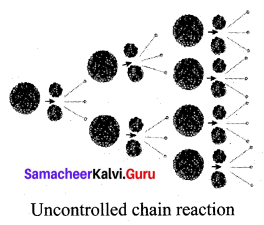Question 2.
Compare the properties of Alpha, Beta and Gamma radiations.

 Properties α rays β rays γ rays What are they? Helium nucleus (2He4) consisting of two protons and two neutrons. They are electrons (−1e0), basic elementary particle in all atoms. They are electromagnetic waves consisting of photons. Charge Positively charged particles. Charge of each alpha particle = +2e Negatively charged particles. Charge of each beta particle = -e Neutral particles. Charge of each gamma particle = zero Ionising Power 100 time greater than β rays and 10,000 times greater than γ rays Comparatively low Very less ionization power Penetrating power Low penetrating power (even stopped by a thick paper) Penetrating power is greater than that of α rays. They can penetrate through a thin metal foil. They have a very high penetrating power greater than that of β rays. They can penetrate through thick metal blocks. Effect of an electric and magnetic field Deflected by both the fields. (in accordance with Fleming’s left-hand rule) Deflected by both the fields, but the direction of deflection is opposite to that for alpha rays. (in accordance with Fleming’s left-hand rule) They are not deflected by both the fields. Speed Their speed ranges from 1/10 to 1/20 times the speed of light. Their speed can go up to 9/10 times the speed of light. They travel with the speed of light.

Question 3.
What is a nuclear reactor? Explain its essential parts with their functions.
Nuclear reactor: A Nuclear reactor is a device in which the nuclear fission reaction takes place in a self – sustained and controlled manner to produce electricity.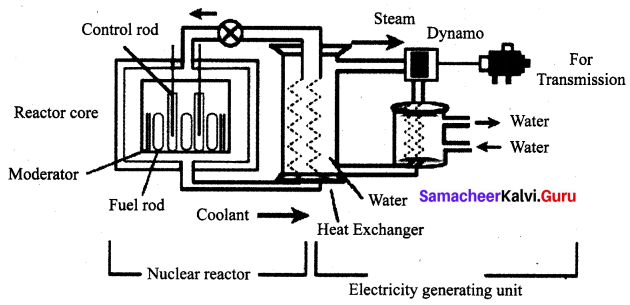Components of a Nuclear Reactor:
The essential components of a nuclear reactor are

• Fuel: A fissile material is used as the fuel. The commonly used fuel material is uranium.
• Moderator: A moderator is used to slow down the high energy neutrons to provide slow neutrons. Graphite and heavy water are commonly used moderators.
• Control rod: Control rods are used to control the number of neutrons in order to have a sustained a chain reaction. Mostly boron or cadmium rods are used as control rods. They absorb the neutrons.
• Coolant: A coolant is used to remove the heat produced in the reactor core, to produce steam. This steam is used to run a turbine in order to produce electricity. Water, air and helium are some of the coolants.
• Protection wall: A thick concrete lead wall is built around the nuclear reactor in order to prevent the harmful radiations from escaping into the environment.

XII. HOT Questions

Question 1.
Mass number of a radioactive element is 232 and its atomic number is 90. When this element undergoes certain nuclear reactions, it transforms into an isotope of lead with a mass number 208 and an atomic number 82. Determine the number of alpha and beta decay that can occur.
Mass number A = 232
Atomic number Z = 90
Daughter element:
Mass number A = 208
Atomic number Z = 82
Difference in mass number = 232 – 208 = 24
Difference in atomic number
= 90 – 82 = 8
Atomic number of α = 2
Atomic number of β = -1
Mass number of α = 4
Mass number of β = 0
Difference in mass number in transformations
= 24
Number of a decays = 244 = 6
Difference in atomic number = 8
ΔZ = 6α + 4β
= 6(2) + 4(-1)
= 12 – 4
= 8
∴ Number of β decays = 4
∴ Number of α decays = 6
∴ Number of β decays = 4

Question 2.
‘X – rays should not be taken often’. Give the reason.

• Radiation does involve in X – rays tests and isotope scans (in nuclear medicine) are too low to cause immediate hazardous effects.
• If should be taken often, X – ray radiation from medical examinations though slightly increases one’s risk for cancer which can occur year or decades after X-ray exposure.

Question 3.
Cell phone towers should be placed far away from the residential area. why?

1. Living near a cell phone tower is not healthy. There is multiple health risks associated with living near a cell phone tower.
2. Cell phone towers communicate by use pulsed microwave signals (radiofrequency radiation) with each other.
3. That is the reason cell phone towers should be placed far away from the residential area.

### Samacheer Kalvi 10th Science Nuclear Physics Additional Questions

I. Choose the best Answer.

Question 1.
Radium was discovered by _____.
(a) Marie curie
(b) Irene curie
(c) Henri Becquerel
(d) F. Joliot.
(a) Marie Curie

Question 2.
How many radioactive substances discovered so far?
(a) 83
(b) 92
(c) 43
(d) 29
(d) 29

Question 3.
The SI unit of Radioactivity is _____.
(a) Curie
(b) Rutherford
(c) Becquerel
(d) Roentgen (R).
(c) Becquerel

Question 4.
(a) increases with increase in temperature
(b) increases with increase in pressure
(c) depends on the number of electrons
(d) purely a nuclear phenomenon.
(d) purely a nuclear phenomenon

Question 5.
Which of the following processes is a spontaneous process?
(c) Photoelectric effect
(d) Collisions

Question 6.
The charge of the β rays _____.
(a) 2e
(b) 0
(c) -e
(d) none of these.
(c) -e

Question 7.
The charge of the γ rays _____.
(a) 2e
(b) 0
(c) -e
(d) none of these.
(b) 0

Question 8.
The atomic number of the elements that exhibit artifical radioactivity is:
(a) more than 82
(b) more than 83
(c) less than 83
(d) less than 82
(c) less than 83

Question 9.
Arrange α, β, γ rays in the increasing order of their ionizing power.
(a) α, β, γ
(b) β, α, γ
(c) γ, β, α
(d) γ, α, β.
(c) γ, β, α

Question 10.
Which produces a charge of 2.58 × 10-4 Coulomb in 1 Kg of air?
(a) Curie
(b) Becquerel
(c) Rutherford
(d) Roentgen
(d) Roentgen

Question 11.
Ionising power of the γ rays _____.
(a) Comparatively very high ionization power
(b) 100 times greater than the α rays
(c) 100 times greater than the β rays
(d) Comparatively very less ionization power.
(d) Comparatively very less ionization power.

Question 12.
Ionization power maximum for _____.
(a) neutrons
(b) α particles
(c) γ rays
(d) β particles.
(b) α particles

Question 13.
Charge of gamma particle is:
(a) +2e
(b) -e
(c) Zero
(d) +1e
(c) Zero

Question 14.
Which has low penetrating power?
(a) α rays
(b) γ rays
(c) β rays
(d) X rays.
(a) α rays

Question 15.
In β – decay _____.
(a) atomic number decreases by one
(b) the mass number decreases by one
(c) proton number remains the same
(d) neutron number decreases by one.
(d) neutron number decreases by one

Question 16.
In which decay the energy level of the nucleus changes:
(a) α – decay
(b) β – decay
(c) γ – decay
(d) neutron decay
(c) γ – decay

Question 17.
In γ – decay _____.
(a) atomic number decreases by one
(b) there is no change in atomic and mass number
(c) energy only changes in the decay process
(d) both (b) and (c).
(d) both (b) and (c).

Question 18.
The unit of decay constant is _____.
(a) no unit
(b) second
(c) second-1
(d) curie.
(c) second-1

Question 19.
The range of temperature required for nuclear fusion is from:
(a) 107 to 109 K
(b) 10-9 to 10-7 K
(c) 105 to 109
(d) 105 to 107 K
(a) 107 to 109 K

Question 20.
1 Rd is equal to _____.
(a) 106 decay / second
(b) 1 decay / second
(c) 3.7 × 1010 becquerel
(d) 1.6 × 1012 decay / second.
(a) 106 decay / second

Question 21.
An element ZXA successively undergoes three α decays and four β decays and gets converted an element Y are respectively _____.
(a) Z−6YA−12
(b) Z+2YA−12
(c) Z−2YA−12
(d) Z−10YA−12.
(c) Z−2YA−12

Question 22.
In the nuclear reaction 88Ra226 → X + 2He4 X is:
(a) 90Th234
(b) 91Pa234
(c) 86Rn222
(d) 88Rn226
(d) 88Rn226

Question 23.
Which one of the following is used in the treatment of skin diseases _____.
(a) Na24
(b) I31
(c) Fe59
(d) P32.
(d) P32.

Question 24.
Anaemia can be diagnosed by _____.
(a) 15P31
(b) 15P32
(c) 26P59
(d) 11P24.
(c) 26P59

Question 25.
Which is used as a coolant?
(a) Graphite
(b) Liquid sodium
(c) Boron
(b) Liquid sodium

Question 26.
The energy released per fission is _____.
(a) 220 MeV
(b) 300 MeV
(c) 250 MeV
(d) 200 MeV.
(d) 200 MeV.

Question 27.
In the reaction 1N14 + 0n1 → X + 1H1 X is:
(a) 15P30
(b) 6C14
(c) 6C12
(d) 11Na23
(c) 6C12

Question 28.
Natural uranium consists of _____.
(a) 99.72 % of U-238
(b) 0.28 % of U-238
(c) 0.72 % of U-238
(d) 99.28 % of U-238.
(d) 99.28 % of U-238.

Question 29.
The number of power reactors in India is _____.
(a) 14
(b) 12
(c) 7
(d) 2.
(a) 14

Question 30.
In the nucleus of 11Na23 the number of protons and neutrons are:
(a) 12, 11
(b) 10, 12
(c) 11, 12
(d) 11, 23
(c) 11, 12

Question 31.
The moderator used in nuclear reactor is _____.
(b) boron carbide
(c) heavy water
(d) uranium (92U235).
(c) heavy water

Question 32.
The first nuclear reactor was built at _____.
(a) Kalpakkam, India
(b) Hiroshima, Japan
(c) Chicago, USA
(d) Trombay, Bombay.
(c) Chicago, USA

Question 33.
Which of the following is used in the treatment of skin cancer?
(d) none of the above

Question 34.
The explosion of an atom bomb is based on the principle of _____.
(a) uncontrolled fission reaction
(b) fusion reaction
(c) controlled fission reaction
(d) none of the above.
(a) uncontrolled fission reaction

Question 35.
The reactor in which no moderator used is _____.
(a) fast breeder reactor
(b) pressurised water reactor
(c) pressurised heavy water reactor
(d) boiled water reactor.
(a) fast breeder reactor

Question 36.
The number of neutrons present in 92U235 is:
(a) 133
(b) 143
(c) 43
(d) 243
(b) 143

Question 37.
In fast breeder, the coolant system used is _____.
(a) heavy water
(b) light water
(c) liquid sodium
(d) boiled water.
(c) liquid sodium

Question 38.
The only reactor in the world which uses U-233 as fuel is _____.
(a) Zerlina
(b) Purnima
(c) Kamini
(d) Tires.
(c) Kamini

Question 39.
The temperature of the interior of Sun is about _____.
(a) 1.4 × 107 K
(b) 108 K
(C) 14 × 107 K
(d) 600 K.
(a) 1.4 × 107 K

Question 40.
Total energy radiated by Sun is about _____.
(a) 3.6 × 1028 Js-1
(b) 3.8 × 1028 Js-1
(c) 3.8 × 1026 Js-1
(d) 3.8 × 1023 Js-1.
(c) 3.8 × 1026 Js-1

II. Fill in the blanks

Question 1.
Cathode rays are discovered by _____.
J.J. Thomson.

Question 2.
Positive rays discovered by _____.
Goldstein.

Question 3.
The chargeless particles are called neutron, it was discovered by _____.

Question 4.
Ernest Rutherford explained that the mass of an atom is concentrated in its central part called _____.
Nucleus.

Question 5.
The radioactive elements emit harmful radiations are ____, ____, ____ rays.
alpha, beta, gamma.

Question 6.
_____ is an spontaneous process.

Question 7.
The element whose atomic number is more than 83 undergoes _____.
spontaneous process.

Question 8.
______ radioactive material is present in the ore of pitchblende.
Uranium.

Question 9.
_____ are the example of artificial (or) man-made radioactive elements.
Boron, Aluminium.

Question 10.
The element whose atomic number is less than 83 undergoes _____.

Question 11.
______ is an controlled manner.

Question 12.
Spontaneous radioactivity is also known as _____.

Question 13.
One Curie is equal to _____ disintegrations per second.
3.7 × 1010

Question 14.
One Rutherford (Rd) is equal to ______ disintegrations per second.
106

Question 15.
The radioactive displacement law is framed by _____.
Soddy and Fajan.

Question 16.
During the α decay process, the atomic number is ______ by 2 and the mass number is decreases by _____.
decreases, 4.

Question 17.
In β-decay the atomic number increases by ____ unit and mass number _____.
One, remains the same.

Question 18.
In α radiation, the charge of each alpha particle is _____.
+2e.

Question 19.
In γ radiation, the charge of each gamma particle is _____.
Zero.

Question 20.
In radioactive radiation, which one is travel with the speed of light _____.

Question 21.
zYA→z−2YA−4+X; Then X is _____.
2He4 (α decay).

Question 22.
zYA→zYA+X; Then X is _____.
γ decay.

Question 23.
The average energy released in each fission process in about _____.
3.2 × 10-11 J.

Question 24.
Fissionable material is a radioactive element, which undergoes fission in a sustained manner when it absorbs a _____.
Neutron.

Question 25.
_____ isotope is used to detect the presence of block in blood vessels and also used for the effective functioning of the heart.
Na24 – Radio sodium.

Question 26.
_____ is used to cure goitre.
Radio Iodine – I131

Question 27.
_____ is used to diagnose anaemia and also to provide treatment for the same.
Radio – iron (Fe59).

Question 28.
Radio cobalt (Co60) and radio gold (Au198) are used in the treatment of _____.
Skin cancer.

Question 29.
_____ are used to sterilize the surgical devices as they can kill the germs and microbes.

Question 30.
The age of the earth, fossils, old paintings and monuments can be determined by _____. technique.

Question 31.
When the body is exposed to about 600 R, it leads to _____.
Death.

Question 32.
Radioactive materials should be kept in a thick – walled container of _____.

Question 33.
_____ is used to remove the heat produced in the reactor core, to produce steam.
Coolant.

Question 34.
The abbreviation of BARC is _____.
Bhabha Atomic Research Centre.

Question 35.
India’s 1st nuclear power station is _____.
Tarapur Atomic Power Station.

Question 36.
The first nuclear reactor built in India was _____.
Apsara.

Question 37.
The total nuclear power operating sites in India is _____.
7

Question 38.
The energy released in a nuclear fission process is about ______
200 Mev.

Question 39.
The number of 0n1 released on an average per fission is _____.
2.5.

Question 40.
A hydrogen bomb is based on the principle of _____.
Nuclear fusion.

III. Match the following

Question 1.

 1. Natural radioactivity (a) 3.7 × 1010 decay/second 2. Artificial radioactivity (b) spontaneous process 3. 1 curie (c) 106 decay/second 4. 1 Rd (Rutherford) (d) induced process

1. (b) spontaneous process
2. (d) induced process
3. (a) 3.7 × 1010 decay / second
4. (c) 106 decay / second

Question 2.

 1. Charge of each α particle (a) γ ray 2. Charge of each β particle (b) +2e 3. Penetration power is maximum (c) α ray 4. Ionisation power is maximum (d) zero

1. (b) +2e
2. (d) zero
3. (a) γ ray
4. (e) α ray

Question 3.

 1. Deuterium (a) −1e0 2. Protium (b) 1H3 3. Tritium (c) 2H4 4. α – decay (d) 1H1 5. β – decay (e) 1H2

1. (e) 1H2
2. (d) 1H1
3. (b) 1H3
4. (c) 2H4
5. (a) −1e0

Question 4.

 1. Uranium core bomb (a) fusion bomb 2. Plutonium core bomb (b) fission bomb 3. Hydrogen bomb (c) Nagasaki 4. Atom bomb (d) Hiroshima

1. (d) Hiroshima
2. (c) Nagasaki
3. (a) fusion bomb
4. (b) fission bomb

Question 5.

 1. Radio iron (Fe59) (a) treatment of skin diseases 2. Radio phosphorous (P32) (b) smoke detector 3. Radio gold (Au198) (c) diagnose anaemia 4. An isotope of Americium (Am241) (d) treatment of skin cancer

1. (c) diagnose anaemia
2. (a) treatment of skin diseases
3. (d) treatment of skin cancer
4. (b) smoke detector

IV. Arrange the following in the correct sequence

Question 1.
Arrange α, β, γ rays in ascending order, on the basis of their penetrating power?
Ascending order:

• Alpha (α)
• Beta (β)
• Gamma (γ)

Question 2.
Arrange in ascending and descending order, on the basis of their Ionisation power.
Alpha (α), Beta (β), Gamma (γ)

1. Ascending order: Gamma (γ), Beta (β), Alpha (α)
2. Descending order: Alpha (α), Beta (β), Gamma (γ)

Question 3.
Arrange in ascending and descending order, on the basis of their biological effect.
Alpha (α), Gamma (γ), Beta (β)

1. Ascending order: Alpha (α), Beta (β), Gamma (γ)
2. Descending order: Gamma (γ), Beta (β), Alpha (α).

V. Numerical Problems

Question 1.
92U238 emits 8α particles and 6β particles. What is the neutron / proton ratio in the product nucleus?
Solution: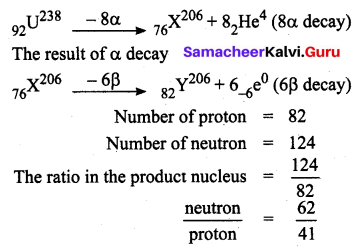Question 2.
The element with atomic number 84 and mass number 218 change to another element with atomic number 84 and mass number 214. The number of α and β particles emitted are respectively?
Solution: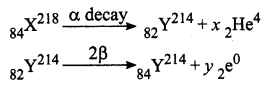Number of alpha decay, x = 1
Number of beta decay, y = 2.

Question 3.
The number of α and β particles emitted in the nuclear reaction 90Th228⟶83Bi12 are respectively.
Solution: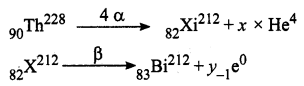Number of α decay, x = 4
Number of β decay, y = 1.

VI. Assertion and Reason Type Questions

(a) If both the assertion and the reason are true and the reason is the correct explanation of the assertion.
(b) If both the assertion and the reason are true, but the reason is not the correct explanation of the assertion.
(c) If Assertion is true, but the reason is false.
(d) If Assertion is false, but the reason is true.
(e) If the Assertion and reason both are false.

Question 1.
Assertion: All the radioactive element are ultimately converted in lead.
Reason: All the elements above lead are unstable.
(c) If Assertion is true, but the reason is false.
Explanation: When they are converted into a lead, the emission is stopped because the nucleus of lead is stable (or lead is most stable elements in radioactive series)

Question 2.
Assertion: Among the alpha, beta and gamma-ray a particle has maximum penetrating power.
Reason: The alpha particle is heavier than beta and gamma rays.
(e) If the Assertion and reason both are false.
Explanation: The penetrating power is maximum in case of gamma rays because gamma rays are electromagnetic radiation of very small wavelength.

Question 3.
Assertion: The ionising power of β – particle is less compared to α – particles but their penetrating power is more.
Reason: The mass of β-particle is less than the mass of α-particle
(b) If both the assertion and the reason are true, but the reason is not the correct explanation of the assertion.
Explanation: β – particle being emitted with very high speed compared to α – particle. Due to this reason, their loss of energy is very slow and they can penetrate the medium through a sufficient depth.

Question 4.
Assertion: Neutrons penetrate matter more readily as compared to protons.
Reason: Neutrons are slightly more massive than protons.
(b) If both the assertion and the reason are true, but the reason is not the correct explanation of the assertion.
Explanation: Neutron is about 0.1 % more massive than a proton. But the unique thing about the neutron is that while it is heavy, it has no charge (it is neutral). This lack of charge gives it the ability to penetrate matter without interacting as quickly as the beta particles or alpha particles.

Question 5.
Assertion: zXA undergoes a decays and the daughter product is z−2YA−4
Reason: In α – decay, the mass number decreases by 4 and atomic number decreases by.
(a) If both the assertion and the reason are true and the reason is the correct explanation of the assertion.
Explanation: zXA⟶z−2XA−4+2He4 (α decay)

Question 6.
Assertion: Moderator is used to slowing down the high energy neutrons to provide slow neutrons.
Reason: Cadmium rods are used as control rods.
(b) If both the assertion and the reason are true, but the reason is not the correct explanation of the assertion.
Explanation: Graphites and heavy water are commonly used moderators. This helps in moderator to slow down the fast neutrons.

Question 7.
Assertion: Alpha, beta and gamma radiations are emitted.
Reason: Nuclear fission process can be performed at room temperature.
(a) If both the assertion and the reason are true and the reason is the correct explanation of the assertion.
Explanation: At room temperature, the nuclear fission process can perform breaking up of heavier nucleus into two smaller nuclei. In this process to emitted the alpha, beta and gamma radiations.

Question 8.
Assertion: An enormous amount of energy is released which is called stellar energy.
Reason: Fusion reaction that takes place in the cores of the Sun and other stars.
(a) If both the assertion and the reason are true and the reason is the correct explanation of the assertion.
Explanation: The stars like our Sun emit a large amount of energy in the form of light and heat. This energy is termed as the stellar energy.

Question 9.
Assertion: Artificial radioactivity is a controlled process.
Reason: It is a spontaneous process – natural radioactivity.
(b) If both the assertion and the reason are true, but the reason is not the correct explanation of the assertion.
Explanation: Artificial radioactivity is a controlled process. It is an induced process and man-made radioactivity.

Question 10.
Assertion: Gamma rays, penetrates through materials most effectively.
Reason: Gamma rays, which have the shortest wavelengths of all electromagnetic radiation.
(a) If both the assertion and the reason are true and the reason is the correct explanation of the assertion.
Explanation: Gamma rays, which have the shortest wavelengths of all electromagnetic radiation. This is a reason they can penetrate through materials most effectively.

VII. Answer the following questions

Question 1.
The phenomenon of nuclear decay of certain elements with the emission of radiations like alpha, beta, and gamma rays is called ‘radioactivity’.

Question 2.
By whom radioactivity is detected in pitchblende?
Marie curie and Purie curie.

Question 3.
The phenomenon by which even light elements are made radioactive, by artificial or induced methods, is called ‘Artificial radioactivity’ or ‘Man – made radioactivity’.

Question 4.
Define ‘One curie’.
It is defined as the quantity of a radioactive substance which undergoes 3.7 × 1010 disintegrations in one second. This is actually close to the activity of 1 g of radium 226.
Curie = 3.7 × 1010 disintegrations per second.

Question 5.
In which elements artifical radioactivity is induced?
Boron and aluminum

Question 6.
What is alpha decay (α decay)? give an example.
A nuclear reaction in which an unstable parent nucleus emits an alpha particle and forms a stable daughter nucleus is called ‘alpha decay’.
E.g. Decay of uranium (U238) to thorium (Th234) with the emission of an alpha particle.
92U238→90Th234+2He4 (α – decay).

Question 7.
What is beta decay (β decay)? Give an example?
A nuclear reaction, in which an unstable parent nucleus emits a beta particle and forms a stable daughter nucleus, is called ‘beta decay’.
E.g. Beta decay of phosphorous.
15P32→16S32+−1e0 (β – decay)

Question 8.
What is gamma decay (γ decay)?
In a γ – decay, only the energy level of the nucleus changes. The atomic number and mass number of the radioactive nucleus remain the same.

Question 9.
State the value of Roentgen in terms of Coulomb.
Roentgen = 2.58 × 10-4 Coulomb in / kg of air.

Question 10.
Define ‘nuclear fission’ Give an example.
The process of breaking (splitting) up of a heavier nucleus into two smaller nuclei with the release of a large amount of energy and a few neutrons are called ‘nuclear fission’.
E.g. Nuclear fission of a uranium nucleus (U235)
92U235+0n1→56Ba141+36Kr92+30n1+Q( energy )

Question 11.
Define ‘Nuclear fusion’ Give an example.
The process in which two light nuclei combine to form a heavier nucleus is termed as ‘Nuclear fusion’.
E.g. 1H2+1H2→2He4+Q( Energy )

Question 12.
Write down the types of the nuclear reactor.
Breeder reactor, fast breeder reactor, pressurized water reactor, pressurized heavy water reactor, boiling water reactor, water – cooled reactor, gas – cooled reactor, fusion reactor and thermal reactor are some types of nuclear reactors, which are used in different places worldwide.

Question 13.
What is the safe limit of receiving radioactive radiations?
100 m R per week

VIII. Answer in the details:

Question 1.
Explain the principle and working of an atom bomb?
Atom bomb:
(i) The atom bomb is based on the principle of the uncontrolled chain reaction. In an uncontrolled chain reaction, the number of neutrons and the number of fission reactions multiply almost in a geometrical progression.

(ii) This releases a huge amount of energy in a very small time interval and leads to an explosion.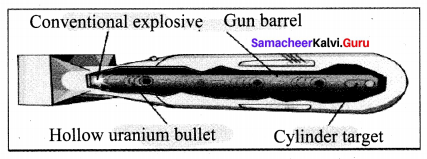Structure:
(i) An atom bomb consists of a piece of fissile material whose mass is subcritical. This piece has a cylindrical void.

(ii) It has a cylindrical fissile material which can fit into this void and its mass is also subcritical. When the bomb has to be exploded, this cylinder is injected into the void using a conventional explosive.

(iii) The two pieces of fissile material join to form the supercritical mass, which leads to an explosion. During this explosion, a tremendous amount of energy in the form of heat, light and radiation is released.

(iv) A region of very high temperature and pressure is formed in a fraction of a second along with the emission of hazardous radiation like y rays, which adversely affect the living creatures. This type of atom bombs was exploded in 1945 at Hiroshima and Nagasaki in Japan during World War II.

Question 2.
State and define the units of radioactivity.
Curie : It is the traditional unit of radioactivity. It is defined as the quantity of a radioactive substance which undergoes 3.7 × 1010 disintegrations in one second. This is actually close to the activity of lg of radium 226. 1 curie = 3.7 × 1010 disintegrations per second.

Rutherford (Rd) : It is another unit of radioactivity. It is defined as the quantity of a radioactive substance, which produces 106 disintegrations in one second.
1 Rd = 106 disintegrations per second.

Becquerel (Bq) : It is the SI unit of radioactivity is becquerel. It is defined as the quantity of one disintegration per second.

Roentgen (R) : It is the radiation exposure of γ and x-rays is measured by another unit called roentgen. One roentgen is defined as the quantity of radioactive substance which produces a charge of 2.58 × 10-4 coulomb in 1 kg of air under standard conditions of pressure, temperature and humidity.

Question 3.
Write down the features of nuclear fission and nuclear fusion.

 Nuclear Fission Nuclear Fusion 1. The process of breaking up (splitting) of a heavy nucleus into two smaller nuclei is called ‘nuclear fission’. 1. Nuclear fusion is the combination of two lighter nuclei to form a heavier nucleus. 2. Can be performed at room temperature. 2. Extremely high temperature and pressure are needed. 3. Alpha, beta and gamma radiations are emitted. 3. Alpha rays, positrons, and neutrinos are emitted. 4. Fission leads to emission of gamma radiation. This triggers the mutation in the human gene and causes genetic transform diseases. 4. Only light and heat energy are emitted.

Question 4.
Write down the medical and industrial application of radioisotopes?

1. Radio sodium (Na24) is used for the effective functioning of the heart.
2. Radio – Iodine (I131) is used to cure goitre.
3. Radio – Iron is (Fe59) is used to diagnose anaemia and also to provide treatment for the same.
4. Radio Phosphorous (P32) is used in the treatment of skin diseases.
5. Radio Cobalt (Co60) and radio – gold (Au198) are used in the treatment of skin cancer.
6. Radiations are used to sterilize the surgical devices as they can kill the germs and microbes.
7. Radio cobalt (Co60) and radio – gold (Au198) are used in the treatment of skin cancer.
8. Radiations are used to sterilize the surgical devices as they can kill the germs and microbes.

Question 5.
Write a note about stellar energy.
The stars like our Sun emit a large amount of energy in the form of light and heat. This energy is termed as the stellar energy. Where does this high energy come from? All-stars contain a large amount of hydrogen. The surface temperature of the stars is very high which is sufficient to induce fusion of the hydrogen nuclei.

Fusion reaction that takes place in the cores of the Sun and other stars results in an enormous amount of energy, which is called as ‘stellar energy’. Thus, nuclear fusion or thermonuclear reaction is the source of light and heat energy in the Sun and other stars.

IX. Additional HOT Questions

Question 1.
Why is neutron so effective as bombarding particle?
A neutron carries no charge. It easily penetrates even a heavy nucleus without being repelled or attracted by nucleus and electrons. So it serves as an ideal projectile for starting a nuclear reaction.

Question 2.
Is there any difference between electron and a beta particle.
Basically, there is no difference between an electron and a beta particle. β particle is the name given to an electron emitted from the nucleus.

Question 3.
Why are the control rods made of cadmium?
Cadmium has high cross – section for the absorption of neutrons.

Question 4.
Name two radioactive elements that are not found in observable quantities why is it so?
Tritium and Plutonium are two radioactive elements that are not found in observable quantities in the universe.
It is because half-life period of each of two elements is very short compared to the age of the universe.

## How to Prepare using Samacheer Kalvi 10th Science Chapter 6 Nuclear Physics Notes PDF?

Students must prepare for the upcoming exams from Samacheer Kalvi 10th Science Chapter 6 Nuclear Physics Notes PDF by following certain essential steps which are provided below.

• Use Samacheer Kalvi 10th Science Chapter 6 Nuclear Physics notes by paying attention to facts and ideas.
• Pay attention to the important topics
• Refer TN Board books as well as the books recommended.
• Correctly follow the notes to reduce the number of questions being answered in the exam incorrectly
• Highlight and explain the concepts in details.

## Frequently Asked Questions on Samacheer Kalvi 10th Science Chapter 6 Nuclear Physics Notes

#### How to use Samacheer Kalvi 10th Science Chapter 6 Nuclear Physics Notes for preparation??

Read TN Board thoroughly, make separate notes for points you forget, formulae, reactions, diagrams. Highlight important points in the book itself and make use of the space provided in the margin to jot down other important points on the same topic from different sources.

#### How to make notes for Samacheer Kalvi 10th Science Chapter 6 Nuclear Physics exam?

Read from hand-made notes prepared after understanding concepts, refrain from replicating from the textbook. Use highlighters for important points. Revise from these notes regularly and formulate your own tricks, shortcuts and mnemonics, mappings etc.
Share: